# RBSE Maths Class 10 Chapter 4: Linear Equation and Inequation in Two Variables Important Questions and Solutions

RBSE Class 10 Maths Chapter 4 – Linear Equation and Inequation in Two variables Important questions and solutions are provided here. All these questions have required graphs, along with detailed explanations. The RBSE Class 10 important questions and solutions available at BYJU’S are prepared by the expert faculty to help the students to improve their problem-solving skills.

Chapter 4 of RBSE Class 10 has various methods of finding solutions of given pairs of linear equations and inequalities. For example, checking if the given pair of equations is consistent or inconsistent, using the comparison ratios. Besides, one more approach of solving equations namely, the graphical method is also explained in detail. The last exercise of this chapter is about drawing graphs for inequalities and solving inequalities by graphical method.

### RBSE Maths Chapter 4: Exercise 4.1 Textbook Important Questions and Solutions

Question 1: On comparing the ratios a1/a2, b1/b2 and c1/c2, find out whether the below pair of linear equations is consistent or inconsistent.

3x – y = 2; 6x – 2y = 4

Solution:

Given pair of linear equations are:

3x – y = 2

⇒ 3x – y – 2 = 0 ….(i)

and 6x – 2y = 4

⇒ 6x – 2y – 4 = 0

⇒ 3x – y – 2 = 0 ….(ii)

Comparing equations (i) and (ii) with a1x + b1y + c1 = 0 and a2x + b2y + c2 = 0,

a1 = 3, b1 = -1, and c1 = -2

a2 = 3, b2 = -1 and c2= -2

a1/a2 = 3/3 = 1

b1/b2 = (-1)/(-1) = 1

c1/c2 = (-2)/(-2) = 1

Therefore, a1/a2 = b1/b2 = c1/c2

Thus, the pair of lines representing the equations are coincident and have infinitely many solutions.

Hence, the given pair of linear equations is consistent.

Question 2: On comparing the ratios a1/a2, b1/b2 and c1/c,2, find out whether the below pair of linear equations is consistent or inconsistent.

2x – 2y = 2; 4x – 4y = 5

Solution:

Given pair of linear equations are:

2x – 2y = 2

⇒ 2x – 2y – 2 = 0

⇒ x – y – 1 = 0 ….(i)

And 4x – 4y = 5

⇒ 4x – 4y – 5 = 0 ….(ii)

Comparing equations (i) and (ii) with a1x + b1y + c1 = 0 and a2x + b2y + c2 = 0,

a1 = 1, b1 = -1, and c1 = -1

a2 = 4, b2 = -4 and c2= -5

a1/a2 = 1/4

b1/b2 = (-1)/(-4) = 1/4

c1/c2 = (-1)/(-5) = 1/5

Therefore, a1/a2 = b1/b2 ≠ c1/c2

Thus, the pair of lines representing the equations are parallel and have no solution.

Hence, the given pair of linear equations is inconsistent.

Question 3: Solve the following pair of linear equations, graphically and find the nature of that solution.

x + y = 3; 3x – 2y = 4

Solution:

Given linear pair of equations are:

x + y = 3

⇒ x + y – 3 = 0 ….(i)

And 3x – 2y = 4

⇒ 3x – 2y – 4 = 0 ….(ii)

Comparing equations (i) and (ii) with a1x + b1y + c1 = 0 and a2x + b2y + c2 = 0,

a1 = 1, b1 = 1, and c1 = -3

a2 = 3, b2 = -2 and c2= -4

a1/a2 = 1/3

b1/b2 = 1/(-2) = -1/2

c1/c2 = (-3)/(-4) = 3/4

Therefore, a1/a2 ≠ b1/b2

Therefore, the given pair of equations is consistent and has a unique solution.

Consider x + y = 3

y = 3 – x

Table for the x and y values of above equation,

 x 0 2 4 y 3 1 -1

Now consider another equation,

3x – 2y = 4

2y = 3x – 4

y = (3/2)x – 2

Table for the x and y values of above equation,

 x 0 2 4 y -2 1 4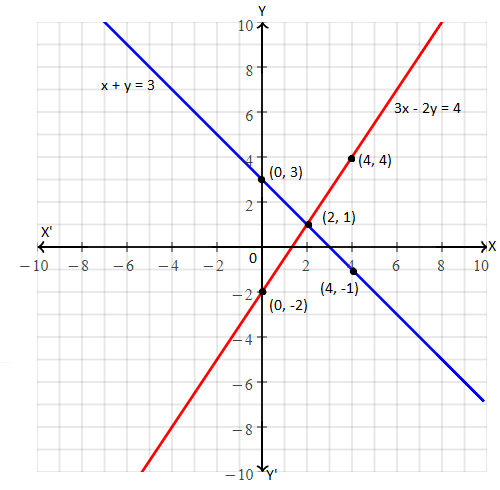The lines representing the given pair of equations intersect each other at (2, 1).

Hence, the solution for the given pair of equations is x = 2 and y = 1.

Question 4: Solve the following pair of linear equations, graphically and find the nature of that solution.

2x – y = 4; x + y = -1

Solution:

Given linear pair of equations are:

2x – y = 4

⇒ 2x – y – 4 = 0 ….(i)

And x + y = -1

⇒ x + y + 1 = 0 ….(ii)

Comparing equations (i) and (ii) with a1x + b1y + c1 = 0 and a2x + b2y + c2 = 0,

a1 = 2, b1 = -1, and c1 = -4

a2 = 1, b2 = 1 and c2= 1

a1/a2 = 2/1 = 2

b1/b2 = -1/1 = -1

c1/c2 = (-4)/1 = -4

Therefore, a1/a2 ≠ b1/b2

Therefore, the given pair of equations is consistent and has a unique solution.

Consider 2x – y = 4

y = 2x – 4

Table for the x and y values of above equation,

 x 0 1 2 4 y -4 -2 0 4

Now consider another equation,

x + y = -1

y = -x – 1

Table for the x and y values of above equation,

 x 0 1 2 4 y -1 -2 -3 -5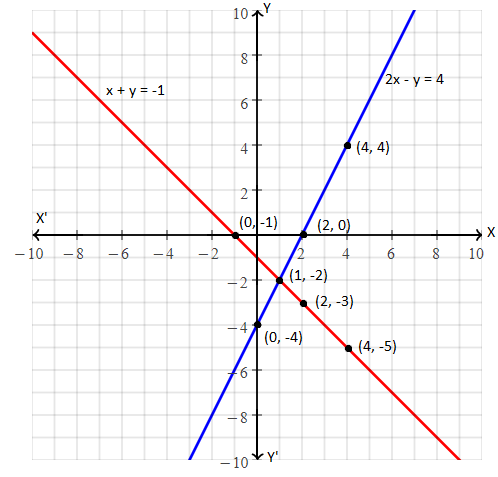The lines representing the given pair of equations intersect each other at (1, -2).

Hence, the solution for the given pair of equations is x = 1 and y = -2.

Question 5: Solve the following linear pairs by graphical method and find coordinates of points where lines represented by them cut y-axis.

2x – 5y + 4 = 0; 2x + y – 8 = 0

Solution:

Given linear pair of equations are:

2x – 5y + 4 = 0 ….(i)

2x + y – 8 = 0 ….(ii)

Comparing equations (i) and (ii) with a1x + b1y + c1 = 0 and a2x + b2y + c2 = 0,

a1 = 2, b1 = -5, and c1 = 4

a2 = 2, b2 = 1 and c2= -8

a1/a2 = 2/2 = 1

b1/b2 = -5/1 = -5

c1/c2 = (4)/(-8) = -1/2

Therefore, a1/a2 ≠ b1/b2

Therefore, the given pair of equations is consistent and has a unique solution.

Consider 2x – 5y + 4 = 0

5y = 2x + 4

y = (⅖)x + (⅘)

Table for the x and y values of above equation,

 x -2 3 8 y 0 2 4

Now consider another equation,

2x + y – 8 = 0

y = -2x + 8

Table for the x and y values of above equation,

 x 0 3 4 y 8 3 0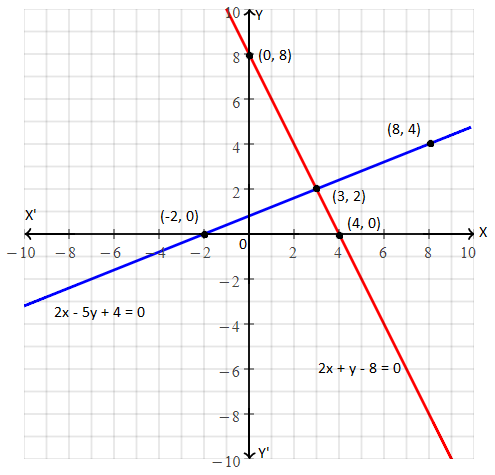The lines representing the given pair of equations intersect y-axis at (0, 8) and (0, 0.8)

Question 6: Solve the following linear pair by graphical method and find coordinates of vertices of triangle, formed by y-axis and lines formed by linear pair.

4x – 5y = 20; 3x + 5y = 15

Solution:

Given linear pair of equations are:

4x – 5y = 20

⇒ 4x – 5y – 20 = 0 ….(i)

And 3x + 5y = 15

⇒ 3x + 5y – 15 = 0 ….(ii)

Comparing equations (i) and (ii) with a1x + b1y + c1 = 0 and a2x + b2y + c2 = 0,

a1 = 4, b1 = -5, and c1 = -20

a2 = 3, b2 = 5 and c2= -15

a1/a2 = 4/3

b1/b2 = -5/5 = -1

c1/c2 = (-20)/(-15) = 4/3

Therefore, a1/a2 ≠ b1/b2

Therefore, the given pair of equations is consistent and has a unique solution.

Consider 4x – 5y = 20

5y = 4x – 20

y = (⅘)x – 4

Table for the x and y values of above equation,

 x 0 5 y -4 0

Now consider another equation,

3x + 5y = 15

5y = -3x + 15

y = (-⅗)x + 3

Table for the x and y values of above equation,

 x 0 5 y 3 0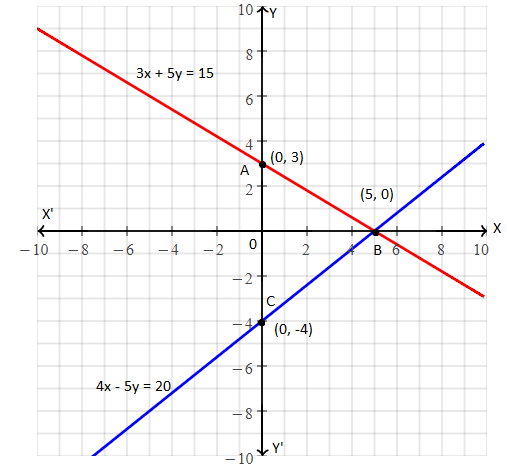Therefore, two lines representing a given pair of equations intersect each other at point (5, 0).

Hence, the solution is x = 5 and y = 0.

Also, A(0, 3), B(5, 0) and C(0, – 4) are coordinates of vertices of ΔABC formed by two straight lines and y – axis

### RBSE Maths Chapter 4: Exercise 4.1 Textbook Important Questions and Solutions

Question 7: Show the solution set of the inequality x ≥ 2.

Solution:

Given inequality is: x ≥ 2

Let use write this equation as x = 2

The graph of x = 2 is the straight line parallel to y-axis and passes through point (2, 0) of x-axis.

The required graph will be obtained by shading the region to the right side of the line as shown below.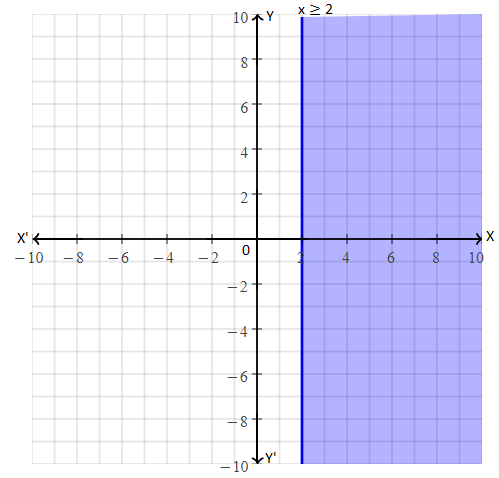Question 8: Show the solution set of the inequality y ≤ -3.

Solution:

Given inequality is: y ≤ -3

Let use write this equation as y = -3

The graph of y = -3 is the straight line parallel to x-axis and passes through point (0, -3) of y-axis.

The required graph will be obtained by shading the region towards the down (i.e. the negative side y-axis) of the line as shown below.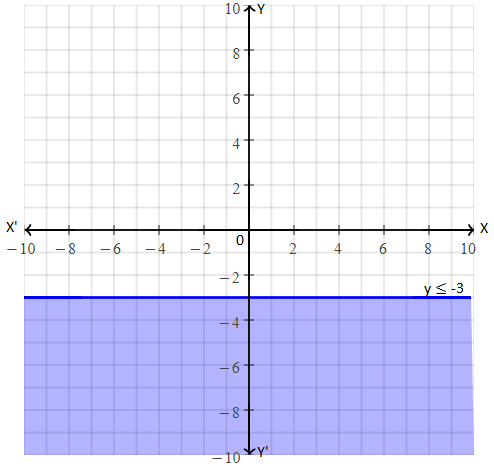Question 9: Show the solution set of the inequality graphically x – 2y < 0.

Solution:

Given inequality is x – 2y < 0

Let x – 2y = 0

2y = x

y = x/2

Table values for x and y to draw the graph:

 x -4 -2 0 2 4 y -2 -1 0 1 2

Plot the above points and join them using a dotted line. Since the equation has less than (<) symbol and shade the region towards the negative x-axis as shown below.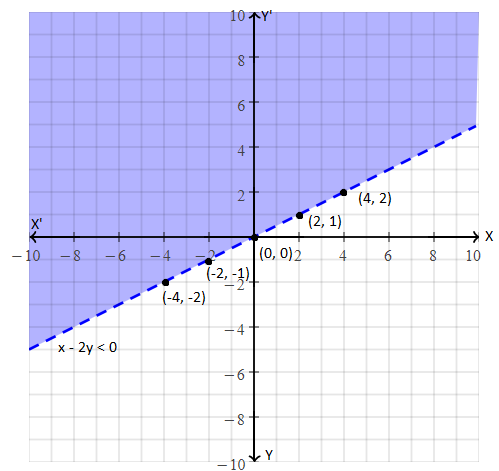Question 10: Show the solution set of the inequality graphically 2x + 3y ≤ 6.

Solution:

Given inequality is 2x + 3y ≤ 6

Let 2x + 3y = 6

3y = -2x + 6

y = -(⅔)x + 2

Table values for x and y to draw the graph:

 x 0 3 y 2 0

Plot the above points and join them using a straight line. Shade the region towards the down of the line as shown below.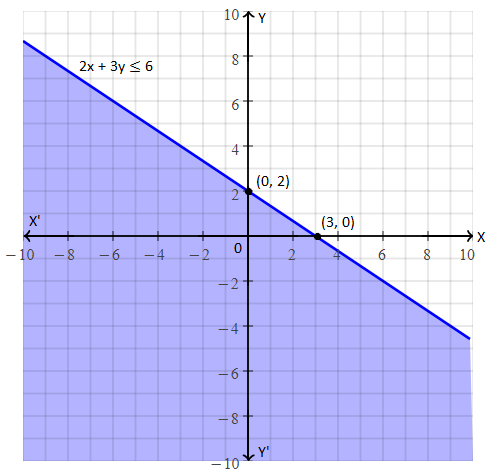Question 11: Solve the following equality by graphical method.

|x| ≤ 3

Solution:

Given inequality is |x| ≤ 3

By removing inequality,

⇒ -3 ≤ x ≤ 3

⇒ x ≤ 3 ….(i)

And x ≥ -3 ….(ii)

From (i),

x = 3

The graph of x = 3 is the straight line parallel to the y-axis on the right side.

From equation (ii),

x = -3

The graph of x = -3 is the straight line parallel to the y-axis on the left side.

Let us draw the graph of both the equations as shown below.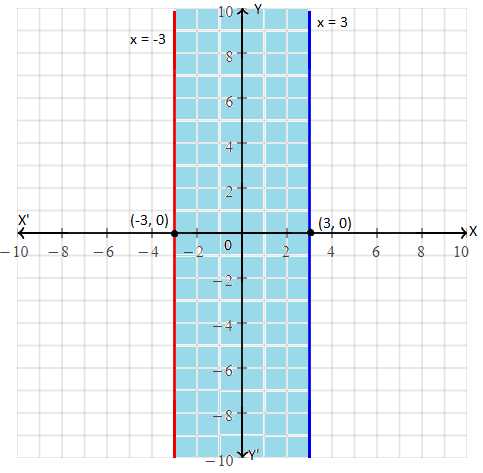Question 12: Solve the following equality by graphical method.

3x – 2y ≤ x + y – 8

Solution:

Given inequality is 3x – 2y ≤ x +y – 8

By removing inequality,

3x – 2y = x + y – 8

⇒ 3x – x – 2y – y = -8

⇒ 2x – 3y = -8

Substituting x = 0,

2 x o – 3y = -8

-3y = -8

y = 8/3

The point is ( 0, 8/3).

Substituting y = 0,

2x – 3 x 0 = -8

x = -4

The point is (-4, 0).

Let us draw the graph from these two points as shown below.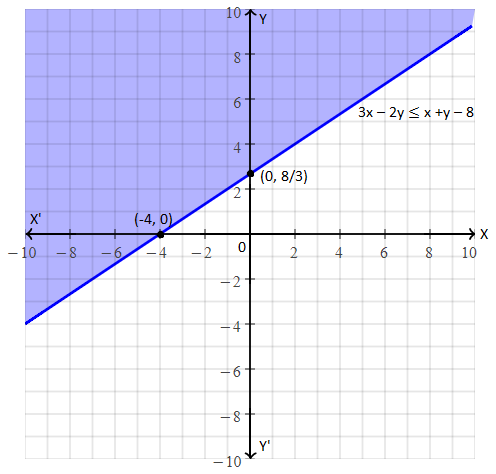Therefore, inequality 3x – 2y ≤ x + y – 8 is not satisfied by origin (0, 0).

⇒ 3 x 0 – 2 x 0 ≤ 0 + 0 – 8, not true.

Hence, the shaded region opposite to origin from line (above the line) will be the required solution set.

Question 13: Solve the following equality by graphical method.

|x – y| ≥ 1

Solution:

Given inequality is |x – y| ≥ 1

By removing modulus,

x – y ≥ 1

And x – y ≤ -1

Thus, the above inequalities can be written as:

x – y = 1 ….(i)

x – y = -1 ….(ii)

Consider equation (i),

Substituting x = 0,

0 – y = 1

y = -1

Substituting y = 0,

x – 0 = 1

x = 1

The points are (0, -1) and (1, 0)

Now, consider equation (ii),

Substituting x = 0,

0 – 1 = -1

y = 1

Substituting y = 0,

x – 0 = -1

x = -1

The points are (0, 1) and (-1, 0)

Plot all these points on a graph and join them using straight lines as shown below.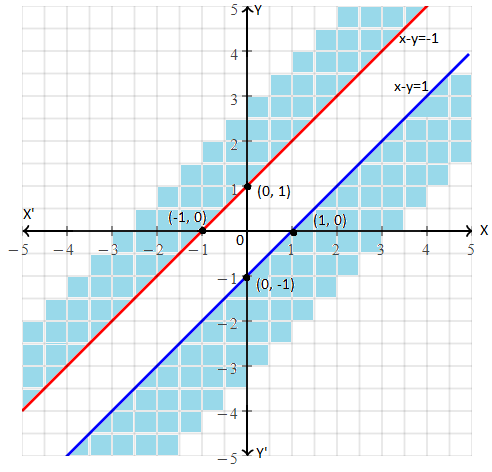Inequality x – y ≥ 1 is not satisfied by the origin (0, 0).

Hence,iIts shaded region will be the region which is opposite to the origin.

And inequality x – y ≤ -1 also not satisfied by the origin (0,0)

Hence, its shaded region will be the region which is opposite to the origin.

### RBSE Maths Chapter 4: Additional Important Questions and Solutions

Question 1: For which value of k, linear pair x + y – 4 = 0; 2x + ky – 3 = 0 have no solution?

(a) 0

(b) 2

(c) 6

(d) 8

Solution:

Given pair of equations are:

x + y – 4 = 0 …..(i)

2x + ky – 3 = 0 ….(ii)

Comparing equations (i) and (ii) with a1x + b1y + c1 = 0 and a2x + b2y + c2 = 0,

a1 = 1, b1 = 1, and c1 = -4

a2 = 2, b2 = k and c2= -3

a1/a2 = 1/2

b1/b2 = 1/k

c1/c2 = (-4)/(-3) = 4/3

Condition for the linear pair having no solution is

a1/a2 = b1/b2 ≠ c1/c2

Thus, ½ = 1/k

k = 2

Question 2: For which value of k, linear pair 3x – 2y = 0 and kx + 5y = 0 have infinite solutions?

(a) 1/2

(b) 3

(c) -5/3

(d) -15/2

Solution:

Given pair of equations are:

3x – 2y = 0 ….(i)

kx + 5y = 0 ….(ii)

Comparing equations (i) and (ii) with a1x + b1y + c1 = 0 and a2x + b2y + c2 = 0,

a1 = 3, b1 = -2, and c1 = 0

a2 = k, b2 = 5 and c2= 0

a1/a2 = 3/k

b1/b2 = -2/5

c1/c2 = 0/0 = 0

Condition for the linear pair having no infinitely many solutions is

a1/a2 = b1/b2 = c1/c2

Thus, 3/k = -⅖

k = (-5/2) (3)

k = -15/2

Question 3: Linear pair kx – y = 2; 6x – 2y = 3 have a unique solution. If

(a) k = 2

(b) k = 3

(c) k ≠ 3

(d) k ≠ 0

Solution:

Given pair of equations are:

kx – y = 2

kx – y – 2 = 0 ….(i)

6x – 2y = 3

6x – 2y – 3 = 0 ….(ii)

Comparing equations (i) and (ii) with a1x + b1y + c1 = 0 and a2x + b2y + c2 = 0,

a1 = k, b1 = -1, and c1 = -2

a2 = 6, b2 = -2 and c2= -3

a1/a2 = k/6

b1/b2 = (-1)/(-2) = 1/2

c1/c2 = (-2)/(-3) = 2/3

Condition for the linear pair having a unique solution is

a1/a2 ≠ b1/b2

Thus, k/6 ≠ ½

k ≠ 6/2

k ≠ 3

Question 4: Inequalities x ≥ 0, y ≥ 0 express corresponding equation to

(a) x-axis

(b) y-axis

(c) x and y-axis

(d) line

Solution:

The graph of x ≥ 0, y ≥ 0 will be as shown below.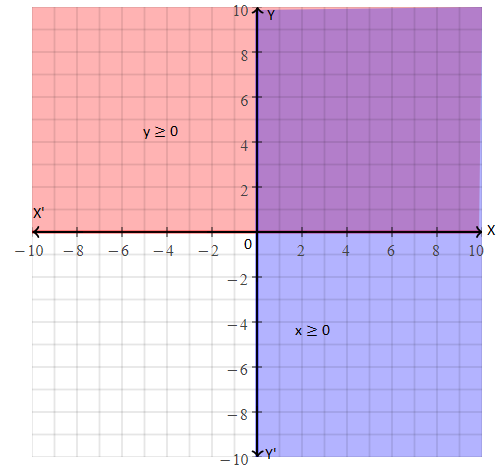Therefore, the given inequalities represent both x and y-axis.

Question 5: Write the number of solutions of the following linear pair.

x + 2y – 8 = 0; 2x + 4y = 16

Solution:

Given pair of equations are:

x + 2y – 8 = 0 ….(i)

2x + 4y = 16

2x + 4y – 16 = 0 ….(ii)

Comparing equations (i) and (ii) with a1x + b1y + c1 = 0 and a2x + b2y + c2 = 0,

a1 = 1, b1 = 2, and c1 = -8

a2 = 2, b2 = 4 and c2= -16

a1/a2 = 1/2

b1/b2 = 2/4 = 1/2

c1/c2 = (-8)/(-16) = ½

a1/a2 = b1/b2 = c1/c2

Therefore, the given pair of linear equations have infinitely many solutions.

Question 6: Solve the following pair of linear equations by graphical method.

3x – 5y = -1

2x – y = -3

Solution:

Given pair of equations are:

3x – 5y = -1 ….(i)

2x – y = -3 ….(ii)

From equation (i),

3x – 5y = -1

5y = 3x + 1

y = (⅗)x + (⅕)

Table for the corresponding x and y values is:

 x -2 3 y -1 2

From equation (ii),

2x – y = -3

y = 2x + 3

Table for the corresponding x and y values is:

 x -2 0 y -1 3

Plot the above points and join using straight lines as shown below.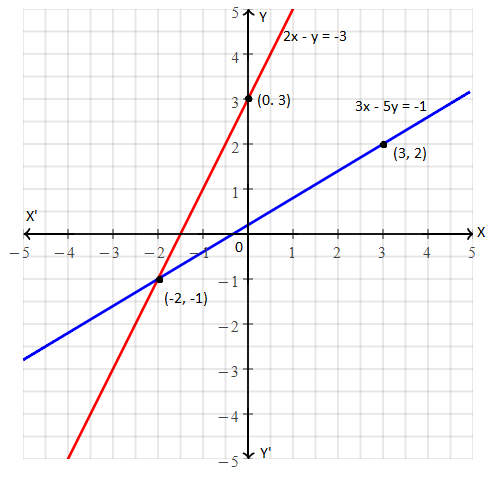The straight lines representing the given pair of equations intersect each other at (-2, -1).

Hence, the solution is x = -2 and y = -1.

Question 7: Solve the following pair of linear equations by graphical method.

2x + y = 6; 2x – y = 2

Thus, find the value of p in the relation 6x + 7y = p.

Solution:

Given pair of equations are:

2x + y = 6 ….(i)

2x – y = 2 ….(ii)

From equation (i),

2x + y = 6

y = -2x + 6

Table for the corresponding x and y values is:

 x -2 3 y -1 2

From equation (ii),

2x – y = 2

y = 2x – 2

Table for the corresponding x and y values is:

 x -2 0 y -1 3

Plot the above points and join using straight lines as shown below.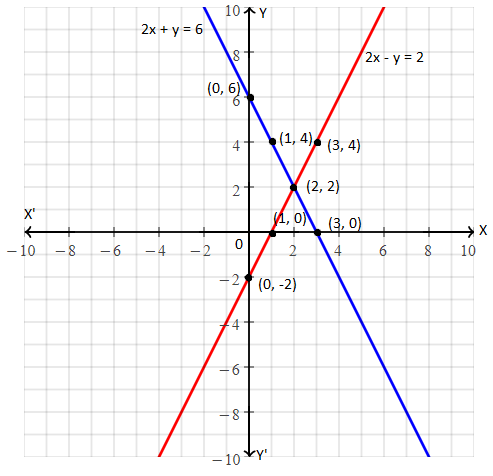The straight lines representing the given pair of equations intersect each other at (2, 2).

Hence, the solution is x = 2 and y = 2.

Given line is, 6x + 7y = p

6(2) + 7(2) = p

12 + 14 = p

Therefore, p = 26